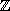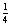Digital & Computing

### Become an OU studentData and processes in computing

Start this free course now. Just create an account and sign in. Enrol and complete the course for a free statement of participation or digital badge if available.

# 2 Forms of data

## 2.1 Numbers

The supermarket example discussed in Section 1 involves various forms of data that a computer may need to handle. Some of these, such as numbers and characters, are simple but fundamental. Other forms of data, such as sequences, involve more complicated structure. In this section, we will introduce sets, which are a variety of data collection that is different from sequences. But first we will look more carefully at numbers and characters.

When developing software we need to distinguish between different sorts of numbers, not least because computers represent and process them differently. Whole numbers (positive, negative or zero) are called integers. We shall use Int to denote the collection (or set) of all integers. In principle, digital computers can represent integers exactly, no matter how large or small. In practice, however, most programming languages place restrictions on the size of an integer (positive or negative) that can be stored. Many texts useinstead of Int.

Sometimes, we need to work with numbers that are not integers. Measured quantities, such as weights or distances, are often presented as non-integer values. More profoundly, even if one starts with whole numbers, some arithmetic processes, such as division or finding a square root, may yield results that are not integers (see Activity 3). The real numbers are a wider collection of numbers used in mathematics. A real number is conveniently thought of as a decimal, such as 435.5218 or –29.333344, but not every real number can be written as a decimal of a finite length. For example, √2 (the square root of 2) and the number(pi) are real numbers that are non-terminating decimals. Such numbers can never be expressed exactly as a finite decimal.is approximately equal to 3.142. You may have met it in calculating the circumference or area of a circle.

Digital computers cannot store real numbers exactly. They need to work with approximations to the real numbers. These approximations may be stored in the computer as floating point numbers. You can get a feeling for the sorts of issues that arise through the following example. Suppose (for the purposes of illustration) that we can only store a real number to an accuracy of four decimal places. Then the number 435.5218 might be stored as 0.4355 × 103. But 435.48336 (a different number) would also be stored as 0.4355 × 103.

If a software application really does need to deal with real numbers, then great care needs to be taken to ensure that the effects of (repeated) approximations are managed in a way that is well understood. This is especially true for safety critical applications, where people's lives may depend upon the behaviour of the software. However, in this course, we shall largely exclude further consideration of real numbers.

### Activity 3

If x and y may each take any integer value, which of the following will always give an integer result and which may give a result that is not an integer? If you are not sure, try to choose values of x and y that do not give integer answers.

• (a) x + y.

• (b) x − y.

• (c) x × y.

• (d) x/y. (x divided by y.)

• (e) x 2.

• (f) √x.

#### Discussion

If x and y are integers, then (a) x + y, (b) x − y and (c) x × y will always be integers. In (e), x 2 means x × x, and if x is an integer then x 2 will be an integer. However, in (d), choosing x = 1 and y = 4 (both integers) givesor 0.25, which is not an integer. (In (d), if y is chosen to be 0, the result is undefined. Software that performs division should always ensure that division by 0 is not attempted.) In (f), choosing x = 8 (for example) gives √8, which is 2.828 (to an accuracy of 4 figures) and is not an integer.